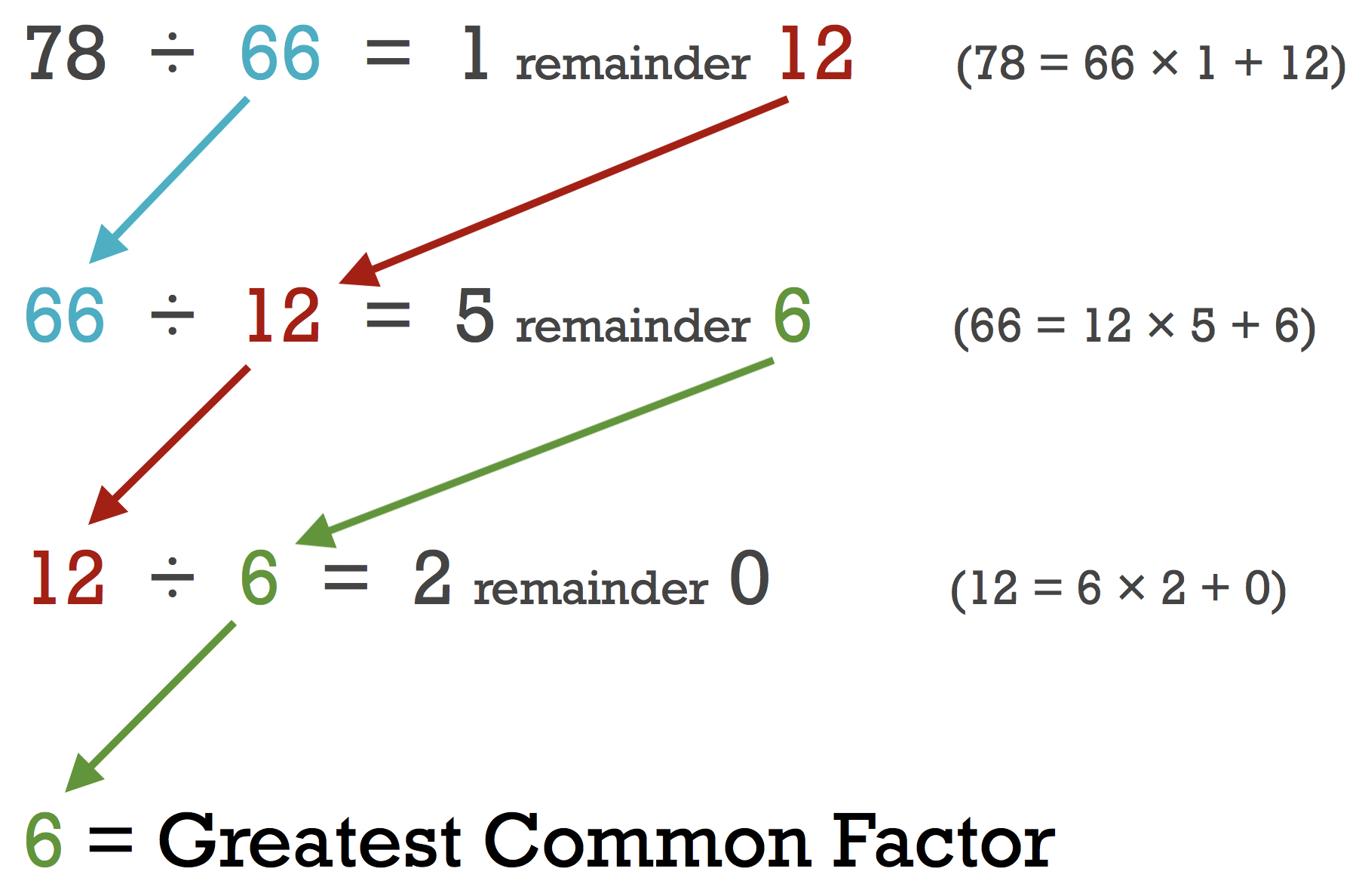# Euclidean Algorithm Calculator

Enter two numbers below to find the greatest common factor between them using Euclid’s algorithm. If you want to find the greatest common factor for more than two numbers, check out our GCF calculator.

## Greatest Common Factor:

5

### Steps to Solve

First, divide the larger number by the smaller number
135÷95=1 remainder40
Divide 95 by 40
95÷40=2 remainder15
Divide 40 by 15
40÷15=2 remainder10
Divide 15 by 10
15÷10=1 remainder5
Divide 10 by 5
10÷5=2 remainder0
Since the remainder is 0, the divisor 5 is the greatest common factor.
Learn how we calculated this below

## How to Use Euclid’s Algorithm to Find the Greatest Common Factor

Euclid’s algorithm defines the technique for finding the greatest common factor of two numbers. The greatest common factor (GCF), also referred to as the greatest common divisor (GCD), is the largest whole number that divides evenly into all numbers in the set.

Euclid’s algorithm is a very efficient method for finding the GCF. To use Euclid’s algorithm, divide the smaller number by the larger number. If there is a remainder, then continue by dividing the smaller number by the remainder.

A ÷ B = Q1 remainder R1
B ÷ R1 = Q2 remainder R2
R1 ÷ R2 = Q3 remainder R3

Continue this process until the remainder is 0 then stop. The divisor in the final step will be the greatest common factor.

For example, find the greatest common factor of 78 and 66 using Euclid’s algorithm.

78 ÷ 66 = 1 remainder 12
66 ÷ 12 = 5 remainder 6
12 ÷ 6 = 2 remainder 0

Thus, the greatest common factor is 6, since that was the divisor in the equation that yielded a remainder of 0.### What if One of the Two Original Numbers is 0?

If either number are 0 then by definition, the larger number is the greatest common factor. In this case it is unnecessary to use Euclid’s algorithm to find the GCF.

If both numbers are 0 then the GCF is undefined.

You’ll probably also be interested in our greatest common factor calculator which can find the GCF of more than two numbers.